1 Simulation Simulation Simulation Computer Model Simulation Simulation

• Slides: 151 시뮬레이션(Simulation) · Simulation의 정의 · Simulation 수행의 주요 단계 및 방법론 · Computer Model의 사용시 고려사항 · Simulation의 형식 · Simulation Software의 바람직한 특성 · Simulation의 장점과 단점2 1. Simulation 의 정의 · A computer-based model used to run experiments on a real system. – – – Typically done on a computer. Determines reactions to different operating rules or change in structure. Can be used in conjunction with traditional statistical and management science techniques.3 2. Simulation 의 주요 단계 Start Run the simulation Define Problem Evaluate results Construct Simulation Model Validation Specify values of variables and parameters Propose new experiment Stop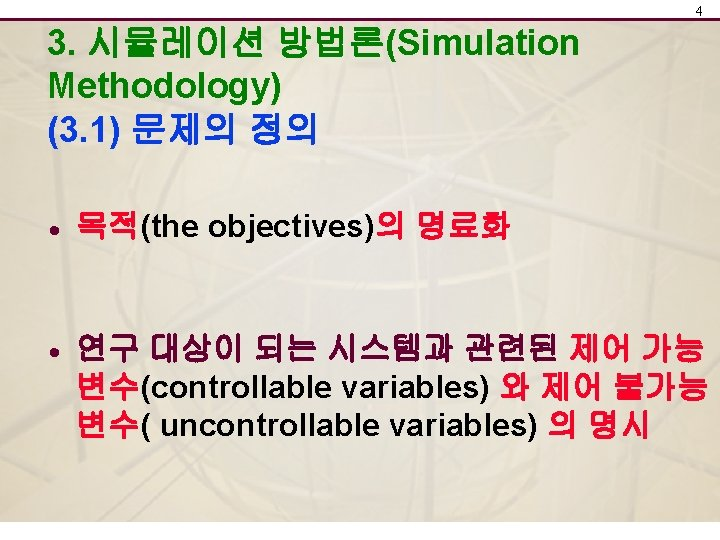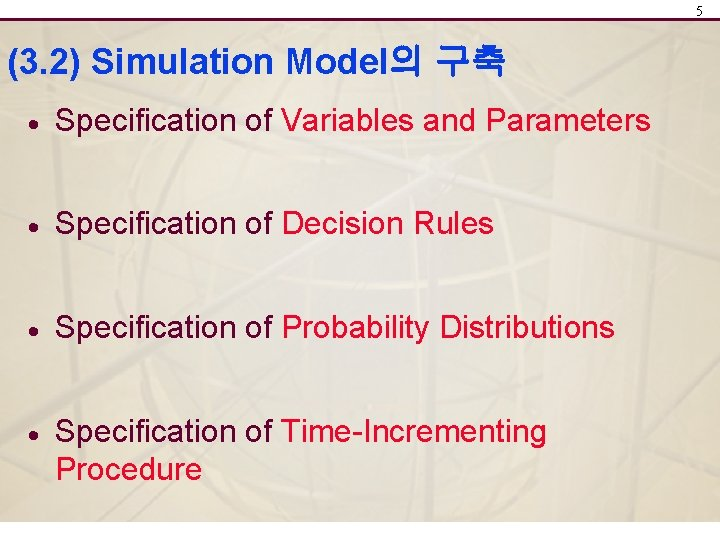5 (3. 2) Simulation Model의 구축 · Specification of Variables and Parameters · Specification of Decision Rules · Specification of Probability Distributions · Specification of Time-Incrementing Procedure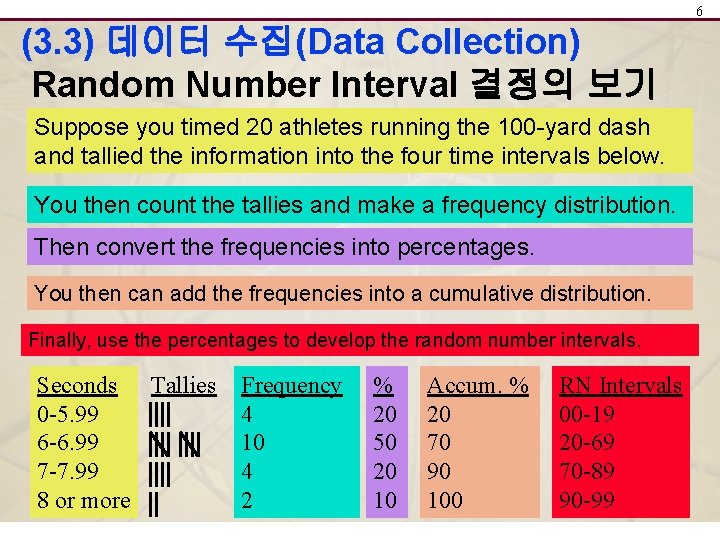6 (3. 3) 데이터 수집(Data Collection) Random Number Interval 결정의 보기 Suppose you timed 20 athletes running the 100 -yard dash and tallied the information into the four time intervals below. You then count the tallies and make a frequency distribution. Then convert the frequencies into percentages. You then can add the frequencies into a cumulative distribution. Finally, use the percentages to develop the random number intervals. Seconds Tallies 0 -5. 99 6 -6. 99 7 -7. 99 8 or more Frequency 4 10 4 2 % 20 50 20 10 Accum. % 20 70 90 100 RN Intervals 00 -19 20 -69 70 -89 90 -997 (3. 4) 결과의 평가 · Conclusions depend on – – · the degree to which the model reflects the real system design of the simulation (in a statistical sense) The only true test of a simulation is how well the real system performs after the results of the study have been implemented.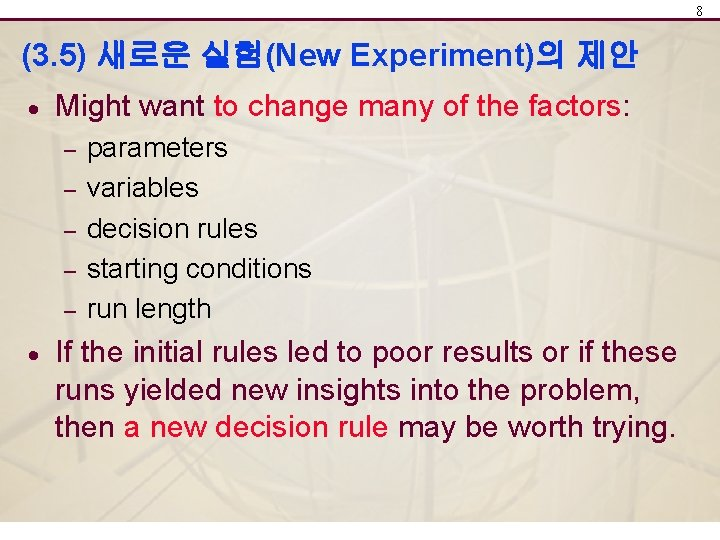8 (3. 5) 새로운 실험(New Experiment)의 제안 · Might want to change many of the factors: – – – · parameters variables decision rules starting conditions run length If the initial rules led to poor results or if these runs yielded new insights into the problem, then a new decision rule may be worth trying.9 4. Computer Model 사용시 고려사항 · Computer language selection · Flowcharting · Coding · Data generation · Output reports · Validation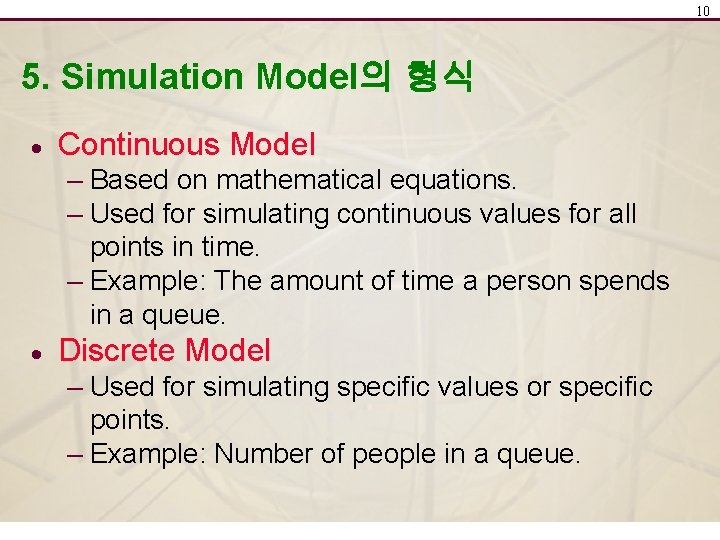10 5. Simulation Model의 형식 · Continuous Model – Based on mathematical equations. – Used for simulating continuous values for all points in time. – Example: The amount of time a person spends in a queue. · Discrete Model – Used for simulating specific values or specific points. – Example: Number of people in a queue.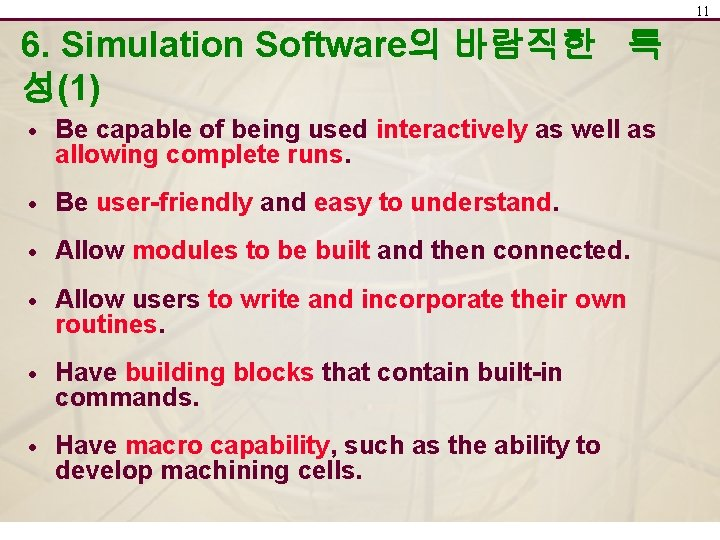11 6. Simulation Software의 바람직한 특 성(1) · Be capable of being used interactively as well as allowing complete runs. · Be user-friendly and easy to understand. · Allow modules to be built and then connected. · Allow users to write and incorporate their own routines. · Have building blocks that contain built-in commands. · Have macro capability, such as the ability to develop machining cells.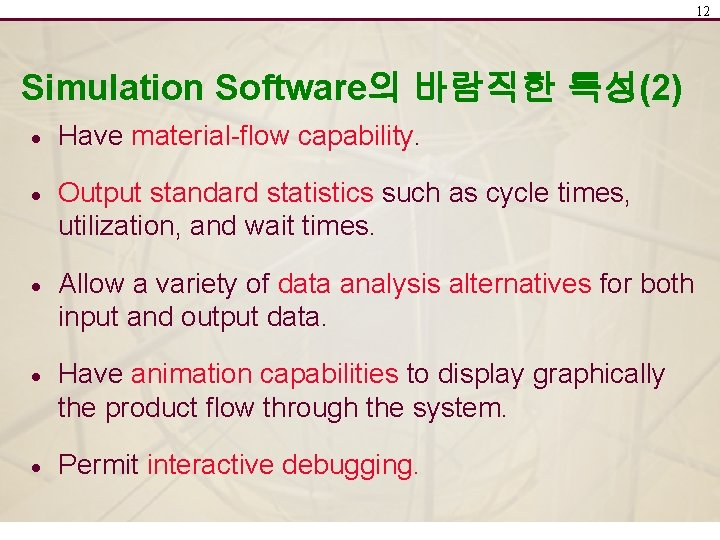12 Simulation Software의 바람직한 특성(2) · Have material-flow capability. · Output standard statistics such as cycle times, utilization, and wait times. · Allow a variety of data analysis alternatives for both input and output data. · Have animation capabilities to display graphically the product flow through the system. · Permit interactive debugging.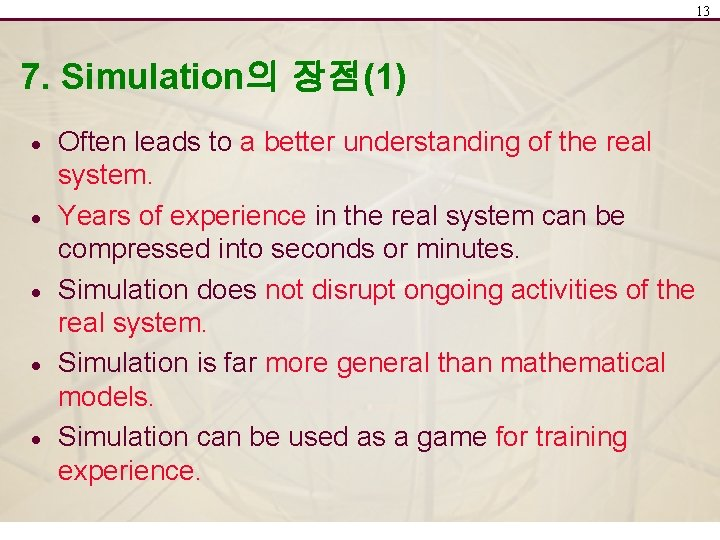13 7. Simulation의 장점(1) · · · Often leads to a better understanding of the real system. Years of experience in the real system can be compressed into seconds or minutes. Simulation does not disrupt ongoing activities of the real system. Simulation is far more general than mathematical models. Simulation can be used as a game for training experience.14 Simulation의 장점 (2) · Simulation provides a more realistic replication of a system than mathematical analysis. · Simulation can be used to analyze transient conditions, whereas mathematical techniques usually cannot. · Many standard packaged models, covering a wide range of topics, are available commercially. · Simulation answers what-if questions.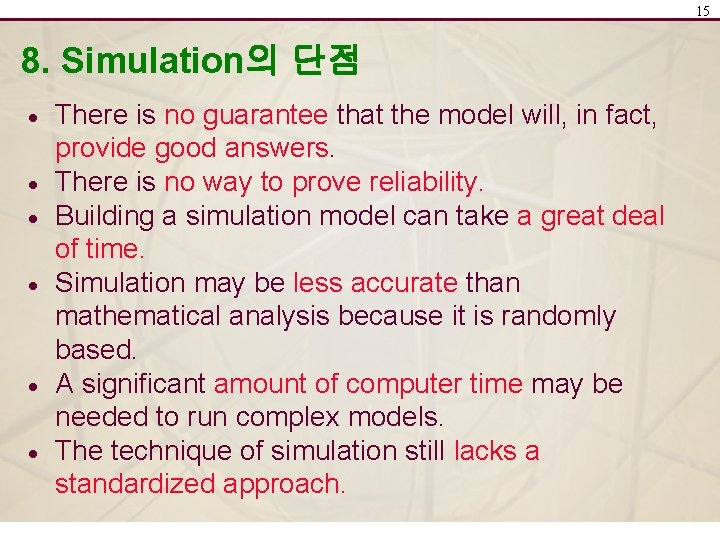15 8. Simulation의 단점 · · · There is no guarantee that the model will, in fact, provide good answers. There is no way to prove reliability. Building a simulation model can take a great deal of time. Simulation may be less accurate than mathematical analysis because it is randomly based. A significant amount of computer time may be needed to run complex models. The technique of simulation still lacks a standardized approach.